Home > INT3 > Chapter 11 > Lesson 11.1.2 > Problem11-20

11-20.
1. For each rational expression below, state any values of the variables that must be excluded (because they will make the denominator zero). Then complete each part. Homework Help ✎

1. Use the fact that (x + 4)2 = (x + 4)(x + 4) to rewrite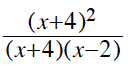. Then look for 1s and simplify.

2. Use the strategy in part (a) to simplify the expression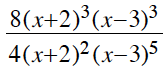.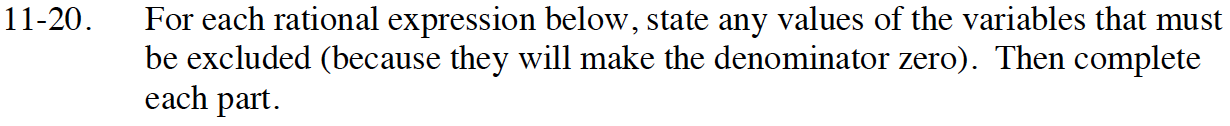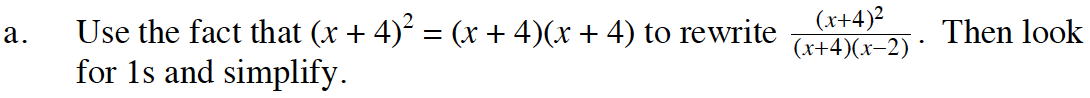Does anything factor out?

x ≠ −4 or 2

$\frac{x+4}{x-2}$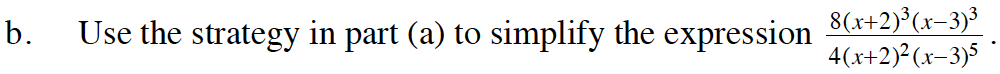Does anything factor out?